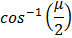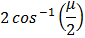# In a laboratory four convex lensesof focal length 2, 4, 6 and 8 cm respectively are available. Two of these lenses from a telescope of length 10 cm and magnifying power 4. The objective and eye lenses are respectively a)b)c)d)## Question ID - 152587 :- In a laboratory four convex lensesof focal length 2, 4, 6 and 8 cm respectively are available. Two of these lenses from a telescope of length 10 cm and magnifying power 4. The objective and eye lenses are respectively a)b)c)d)3537

 (d) Length of tube = 10 cmMagnificationPutting in Eq. (I)Hence,andwill be used.
 A ray of light passes from vacuum into a medium of refractive index, the angle of incidence is found to be twice the angle of refraction. The angle of incidence is a)b)c) 2d)